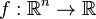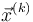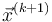# Approximate Newton's method with constant approximate inverse Hessian

## Definition

Approximate Newton's method with constant approximate inverse Hessian refers to a type of approximate Newton's method for optimization of a function of multiple variables where the matrix used as the stand-in for the inverse Hessian is constant across iterations, independent both of the current value of the iterate and of the number of iterations.

## Iterative step

Note that we use parenthesized superscripts to denote iterates and iteration-specific variables, and subscripts to denote coordinates. Parenthesized superscripts do not denote exponents.

Suppose we are applying gradient descent to minimize a function$f:\R^n \to \R$. We will denote the input to$f$ as a vector$\vec{x} = (x_1,x_2,\dots,x_n)$. Given a guess$\vec{x}^{(k)}$, gradient descent computes the next guess$\vec{x}^{(k+1)}$ as follows:$\vec{x}^{(k+1)} = \vec{x}^{(k)} - S \nabla f\left(\vec{x}^{(k)}\right)$

Here,$S$ is intended as a stand-in (an approximation or a bound of some sort) on the inverse matrix of the Hessian matrix of$f$. Unlike the usual Newton's method for optimization of a function of multiple variables, where$S$ would be replaced by the inverse Hessian at the point$\vec{x}^{(k)}$, the matrix$S$ needs to be independent of$\vec{x}^{(k)}$.

## Particular cases

### The one-dimensional version is just gradient descent with constant learning rate

In one dimension, this reduces to gradient descent with constant learning rate, where the constant approximate inverse Hessian is the$1 \times 1$ matrix with entry equal to the constant learning rate. Therefore, the analysis of that problem applies here. In particular, see:

### The general case

Function type Discussion of approximate Newton's method with constant approximate inverse Hessian
quadratic function of multiple variables approximate Newton's method with constant approximate inverse Hessian for a quadratic function of multiple variables
convex function of multiple variables approximate Newton's method with constant approximate inverse Hessian for a convex function of multiple variables